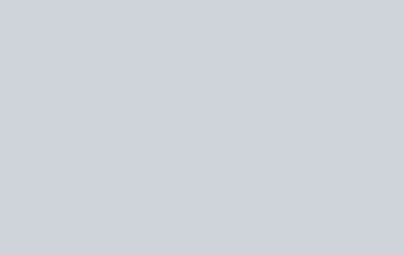In below figure, lines AB and CD intersect at O.
Question:

In below figure, lines AB and CD intersect at O. If ∠AOC + ∠BOE = 70° and ∠BOD = 40°, find ∠BOE and reflex ∠COE?Solution:

Given that

AOC + BOE = 70 and BOD = 40

To find BOE

Here, BOD and AOC an vertically opposite angles

BOD = AOC = 40

Given, ∠AOC + ∠BOE = 70°,

⟹ 40 + BOF = 70

⟹ BOF = 70 – 40

⟹ BOE = 30

AOC and BOC are lines pair of angles

⟹ AOC + COF + BOE = 180

⟹ COE = 180 – 30 – 40

⟹ COE = 110

Hence, Reflex COE = 360 – 110 – 250.